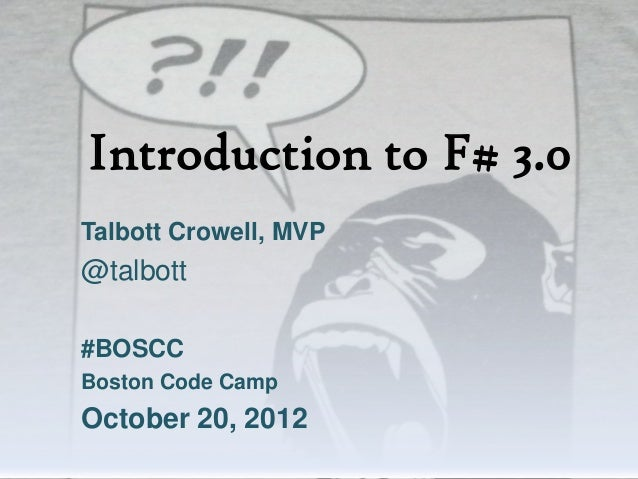Successfully reported this slideshow.
We use your LinkedIn profile and activity data to personalize ads and to show you more relevant ads. You can change your ad preferences anytime.Upcoming SlideShare
Loading in …5
×

# Introduction to F# 3.0

6,497 views

Published on

Talbott Crowell introduces F# 3.0 including function programming basics, units of measure, and the new F# 3.0 feature called Type Providers for analyzing Big Data

Published in: Technology
• Full Name
Comment goes here.

Are you sure you want to Yes No
Your message goes here• Be the first to comment

### Introduction to F# 3.0

1. 1. Introduction to F# 3.0Talbott Crowell, MVP@talbott#BOSCCBoston Code CampOctober 20, 2012
2. 2. What is F#Statically typed, open source functional programming language that uses type inferencing Unique features: Units of Measure Type Providers
3. 3. Static versus DynamicF# is statically typed like: C++, Objective-C, Java, ScalaCompared to dynamically typedlanguages: Python, JavaScript, Erlang, MATLAB
4. 4. Open SourceApache 2.0 licenseFree IDE on Windows from MicrosoftFree IDE on Mac (MonoDevelop)http://blogs.msdn.com/b/fsharpteam/archive/2012/09/24/announcing-the-f-3-0-open-source-code-drop.aspx
5. 5. Functional Programming (FP)Examples of FP languages Erlang, Haskell, Clojure and Scala (JVM)Functions are first class citizens like variables in imperative languagesHigher-order functions functions a parameters or return values
6. 6. Type Inferencinglet x = 5let y = "hello"let z = new Form()let distance = 26.21875<mile>let f x = x + x
7. 7. Immutable by defaultlet x = 5let mutable y = 7y <- 8let z = new Form()z.Show()z.Text <- “Hello”
8. 8. Array, List, and SeqArrays are mutableLists are notSeq (sequences) only store onevalue at a time (lazy)
9. 9. Array, List, and Seqlet set1 = [|1..10|]let set2 = [1..10]let set3 = seq { 1..10 }set1. <- 999 //mutable
10. 10. Units of MeasureFloating point and signed integervalues in F# can have associatedunits of measure, which are typicallyused to indicatelength, volume, mass, and so on.By using quantities with units, youenable the compiler to verify thatarithmetic relationships have thecorrect units, which helps preventprogramming errors.
11. 11. Units of Measure[<Measure>] type mile[<Measure>] type kmlet distance1 = 4.0<mile>let distance2 = 3.0<km>//compile errorlet total = distance1 + distance2
12. 12. Units of Measure[<Measure>] type mile[<Measure>] type kmlet convert_mile2km (a : float<mile>) = a / 0.621371<mile/km>let distance1 = 4.0<mile>let distance1a = convert_mile2km distance1let distance2 = 3.0<km>let total = distance1a + distance2
13. 13. Type ProvidersF# 3.0 introducesType ProvidersData rich programmingfor the Big Data EraEnablesmassive schemaswith strict typing
14. 14. Examples of Type Providers SQL Data Connection OData Service Provider (Netflix) Freebase Hadoop R WMI RegEx
15. 15. Type Provider Demo
16. 16. TryFsharp.org
17. 17. FPish.net
18. 18. Other Resourceshttp://stackoverflow.com/questions/tagged/f%23http://fsharpsamples.codeplex.com/http://fsharp.net
19. 19. Questions?
20. 20. Thank YouFollow me on Twitter @Talbott